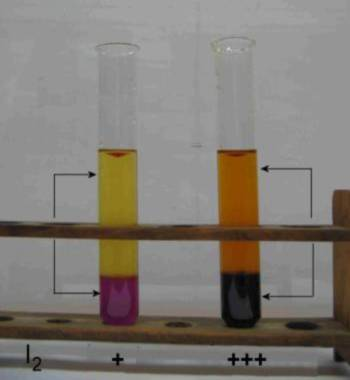Search:

# The heterogeneous equilibrium

## Experiment

Two test tubes contain the same non miscible liquids: Water (at the top) and carbon tetrachloride ($CCl_4$, at the bottom). So we have two liquid phases. The system is heterogeneous. Iodine ($I_2$) is introduced and equilibrium is established. Here are the results:The ratio of the intensities of coloration (therefore the molarities of $I_2$) seems to be constant.

## Interpretation

Let's call the upper water phase $C$ and the lower tetrachloride phase $B$. At the separtion surface between the phases .. - Iodine passes from $C$ to $B$ all the more rapidly than its concentration in $C$ is high: $v_{C \rightarrow B}$ $=$ $k_1 [I_2]_C$   (1) $v_{C \rightarrow B}$ is the rate of passage from $C$ to phase $B$, $[I_2]_C$ is the molarity of iodine in the phase $C$ and $k_1$ is a rate constant depending on the temperature alone. - Iodine passes from $B$ to $C$ all the more rapidly than its concentration in $B$ is high: $v_{B \rightarrow C}$ $=$ $k_2 [I_2]_B$  (2) $v_{B \rightarrow C}$ is the rate of passage from $B$ to phase $C$, $[I_2]_B$ is the molarity of iodine in the phase $B$ and $k_2$ is a rate constant depending on the temperature alone. At the time the two rates become equal, an equilibrium is established: $v_{B \rightarrow C}$ $=$ $v_{C \rightarrow B}$ $k_1 [I_2]_C$ $=$ $k_2[I_2]_B$ $\frac{[I_2]_C}{[I_2]_B}$ $=$ $\frac{k_2}{k_1} = K$ And that confirms that the intensities of coloration (therefore the molarities of $I_2$) are constant!

## Generalisation

When a solute $X$ is in balance between two phases $C$ and $B$, we have: $\frac{[X]_C}{[X]_B}$ $=$ $K$ The ratio of molarities between the two phases called "partition constant" $K$ depends only on the temperature.